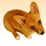# Arcade expression to calculate average

774
6
02-23-2022 08:54 PMOccasional Contributor III

I have a table with five fields for sampling records and a sixth field for a mean/average value to be calculated in.

I have used the Arcade command 'Average' within 'Field Calculator', but find it does not calculate a correct value when 0 values are recorded within some fields.

Average(\$feature.fld0,\$feature.fld1,\$feature.fld2,\$feature.fld3,\$feature.fld4)

Average(1,2,3,0,0) should equal (1+2+3) / 3 = 2, but the value I am getting is (1+2+3+0+0) / 5 = 1.2

What would be the expression to calculate the correct average value when some fields have 0 values (ie. exclude fields with a 0 value from the calculation)?

6 RepliesbyMVP Esteemed Contributor

You  can Arcade the logic from this python example

``````# python... enclose field names in ! eg. !f0!
# example row
f0, f1, f2, f3, f4 = 3, 2, 1, 0, 0

sum([f0, f1, f2, f3, f4])/len([i for i in [f0, f1, f2, f3, f4] if i > 0])
2.0``````

... sort of retired...byMVP Regular Contributor
``````function non_zero_average(values) {
var non_zero = []
for(var i in values) {
var v = values[i]
if(!IsEmpty(v) && v != 0) {
Push(non_zero, v)
}
}
return Average(non_zero)
}
return non_zero_average([1, 2, 3, 0, null])``````

Have a great day!
JohannesbyMVP Honored Contributor

Just to throw another approach on the heap, we can use Filter to simply drop those zeroes and nulls as well.

``````var vals = [1, 2, 3, 0, 0, Null]

function DropEm(value){ DefaultValue(value, 0) != 0 }

Average(Filter(vals, DropEm))``````

It still pales in comparison to the concision of Python, though.

- Josh Carlson
Kendall County GISNew Contributor

Having the same problem and am unable to understand any of the replies above being almost novice in programming, although they make sense to me. My function is as follows:

Average(\$feature.Qrt1_2022, \$feature.Qrt2_2022, \$feature.Qrt3_2022, \$feature.Qrt4_2022)

All I want it to do is don't account for 0 values.byMVP Regular Contributor

Let's look at Josh's answer above you. What it does is the following:

• get an array of values
• define a function with which to filter this array. this function returns true if you want the value to stay in the array. we want a value to stay in the array if it is unequal to zero (!=0). but we also have to account for null values. that's what DefaultValue does: it returns the value if it is not null and returns a default value (0 in this case) if it is null.
• filter the array
• get the average of the filtered array

So, if we apply Josh's answer to your problem (and make the steps more obvious):

``````// get an array of values
var values = [\$feature.Qrt1_2022, \$feature.Qrt2_2022, \$feature.Qrt3_2022, \$feature.Qrt4_2022]

// define a function that we use to filter the array
// returns true (keep value) if value is not null and is unequal to 0
function drop_zero(value) {
var non_null_value = DefaultValue(value, 0)  // value is null? -> value = 0
return non_null_value != 0
}

// filter the array
var filtered_values = Filter(values, drop_zero)

// return the average of the filtered array
return Average(filtered_values)``````

Have a great day!
JohannesNew Contributor

Thank you. This was very helpful.